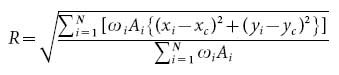## Test 4

2-D flow over a rippled bed

(A. Panizzo)

This benchmark case has been inspired by an experimental work on the study of vortices generated by an oscillatory flow over a rippled bed. The study was carried out by Earnshaw and Greated (1998) at the University of Edinburgh. Results were geared using the PIV technique of the experimental flow fields, aiming at the characterization of the vortex structures caused by the bed shape. Indeed, a solid rippled bed was used, with length, crest to crest, equal to 220 mm, and height, trought to crest, equal to 35 mm (see Figure 1). The ripple shape can be approximated by an arc of radius 190 mm. The following sketch can be used for the computational domain design (measures are in mm).Figure 1. Proposed computational domain.

Three different flow and bed conditions are here proposed for SPH numerical tests:Table 1. Parameters of the oscillatory flow.

Where Ub is the amplitude of the wave velocity (m/s), T is the time period (seconds), a is the amplitude of oscillations (in cm), and lambda is the ripple length. Since it is a 2D motion, there is only a component of vorticity in the z-direction:Eq. 1

In the calculation of the vorticity field, Earnshaw and Greated (1998) suggest to set a cut off value, equal to 10% of the vorticity maximum which is taken to identify all the produced vortices. The circulation is referred to as the scalar function giving the measure of the strength of a vortex, and can be defined as:Eq. 2

If the vorticity (Eq. 1) is calculated using a grid, can be calculated as:Eq. 3

Figure 2 reports values of for the three proposed experimental cases performed by Earnshaw and Greated (1998), as a function of the phase . The line with points refers to case 1, triangles refer to test 2, + refers to test 3.Figure 2. Vortex intensity.

Making reference to the ripple crest as the origin of the xy-plane, the vortex center can be calculated using the following equation (an analogous equation can be written for y):Eq. 4

Analogously, the vortex physical size can be calculated using the following equation:Eq. 5

Figures 3, 4 and 5 report experimental PIV results characterizing the vortex center and radius for the three proposed experimental cases.

#### Suggestions

If you have something to add to this or is there something else that you think should be added, please email me.

#### References

Earnshaw H. C., Greated C. A. (1998) "Dynamics of ripple bed vortices”, Exp. in Fluids, 25, 265-275, Springer-Verlag.